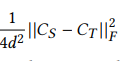# Using Frobenius Norm loss

My model looks like this:

``````def conv_layer(ni,nf,kernel_size=3,stride=1):
return nn.Sequential(
nn.BatchNorm2d(nf,momentum=0.01),
nn.LeakyReLU(negative_slope=0.1,inplace=True)
)

class block1(nn.Module):
def __init__(self,ni):
super().__init__()
self.conv1 = conv_layer(ni,ni//2,kernel_size=1)
self.conv2 = conv_layer(ni//2,ni,kernel_size=3)
self.classifier = nn.Linear(ni*8*4,751)

def forward(self,x):
x = self.conv2(self.conv1(x))
x = x.view(x.size(0),-1)
return self.classifier(x)

class block2(nn.Module):
def __init__(self,ni):
super().__init__()
self.conv1 = conv_layer(ni,ni//2,kernel_size=1)
self.conv2 = conv_layer(ni//2,ni,kernel_size=3)
self.classifier = nn.Linear(ni*8*4,1360)

def forward(self,x):
x = self.conv2(self.conv1(x))
x = x.view(x.size(0),-1)
return self.classifier(x)
``````

I want to take features from conv2 layer of both block1 and block2 and apply forbenius norm loss like this:
X =where Cs denotes features from conv2 layer of block2 and Ct denotes features from conv2 layer of block1.
Covariance isXi,Xj are the features from different images.
I have to take covariance of positive samples only. Then using Hinge loss = max(0,X) I want to add this along my cross entropy loss function.

What is the value of d in above equation?
I didn’t understand your question properly. After getting the values of Cs and Ct, you’ll substitute the values in above equation and get the value of X. Is X your final value as input to Hinge loss?

D is just constant ,don’t consider it. Yeah

Ok, you can write a class of your own inheriting HingeLoss.

``````class loss_criteria(torch.nn.HingeEmbeddingLoss):
def __init__(self,margin=1.0, size_average=True, reduce=True):
super(loss_criteria, self).__init__(margin, size_average, reduce)

def forward(self, cs, ct):
D = 1.0 # constant value
x = ((torch.abs(cs - ct))/(2*D))**2 #formula
hinge_loss = torch.nn.HingeEmbeddingLoss()
y = hinge_loss(x) # sorry, couldn't write the whole correct equation here
return y

criterion = loss_criteria()
criterion(cs,ct)
``````

1 Like

Thanks,How do i extract features from conv2 layer ? Extracting Cs,Ct is the main task

``````model2 = block2()
block2_child = torch.nn.Sequential(*list(model2.children()))[:-1]
conv2_features_from_block2 = block2_child(<pass the input>)
``````

I did it by using forward hooks. They prove to be much efficient.

x = ((torch.abs(cs - ct))/(2*D))**2 #formula

should this be torch.norm(cs - ct, 2) * constant in this case for calculating the norm?